“Probability Theory Should Be Thrown Under A Bus” — Artifical Intelligence Expert, Carlos E. Perez.

We start off by studying Probability Theory and then delve into statistics.

Probability and Statistics are used all the time in Computer Science. Machine learning? It’s probability. Data science? It’s statistics.

### High Level Probability#

Probability provides a way of summarising the uncertainty that comes from our laziness and ignorance. In other words, probability finds out the likeliness that something will happen.

### Discrete Probability#

Discrete probability is a formalisation of probability theory that describes probability for usage in computers from discrete mathematics.

When solving problems with discrete probability, we start with using probability spaces. A probability space is the pairing (S, P) where:

1. S is the sample space of all elementary events X ∈ S. Members of S are called outcomes of the experiment.
2. P is the probability distribution, that is, assigning a real number P(x) to every elementary event X ∈ S such that it’s probability is between 0 and 1 and ∑P(x) = 1

For point 2, P(x) is read as “the probability of X”. The probability must always be between 0 and 1, or often represented as 0% and 100%.

### Example#

Imagine flipping a coin. The probability space is (S, P).The outcome S is S = {H, T} where S can either be Heads or Tails.Therefore the probability isP(H) = P(T) = 1/2The probability for heads is the same as the probability for tails which is the same as a half. In other words, if you flip a coin there is an even chance of it becoming head-side up or tail-side up.

A probability distribution is considered uniform if every outcome is equally as likely.

### An Introduction to Solving Probability Problems#

Many many people including university professors and PhD students cannot solve probability problems. As discussed later in this article the Monty Hall problem is a famous problem and good example of this.

Suppose you’re on a game show, and you’re given the choice of three doors: Behind one door is a car; behind the others, goats. You pick a door, say №1, and the host, who knows what’s behind the doors, opens another door, say №3, which has a goat. He then says to you, “Do you want to pick door №2?” Is it to your advantage to switch your choice?

This question was sent into Voe Savant who at the time had the highest IQ in the world. Voe Savant replied that there is a 2/3rd chance of winning the car if you switch and 1/3rd if you don’t switch.

Thousands of people argued over the Monty Hall problem and many university professors of maths said that math illiteracy was rampant in America because the Monty Hall problem solution suggested was wrong.

This problem appeared in every maths class the following week and thousands of readers, many who have PhDs in maths wrote in to explain that Savant was wrong. Even Paul Erdős, one of the worlds most famous mathematicians, said that Savant was wrong.

Unfortunately for them, Savant was right. This is a simple probability problem that if defined formally can be explained. Many of the mathematicians used their intuition to solve this problem and not follow the steps in solving a probability problem that will be outlined below.

There are a few steps you need to take before you solve a probability problem to prove you fully understand the problem.

### Sample Space#

The sample space is the set which contains all possible outcomes.

So given a coin, the sample space is {heads, tails} as the coin can only land on heads or tails.

### Outcome#

An outcome consists of all the information of an experiment after the experiment has been performed. When you flip a coin and it lands on heads, the outcome is {heads}.

### Probability Space#

The probability space is the sample space but every possible outcome has a probability applied to it. With the coin flip the probability space is {(Heads, 0.5), (Tails, 0.5)}.

The total probability of all probabilities in the probability space must be equal to 1. No single probability can be less than 0 or more than 1.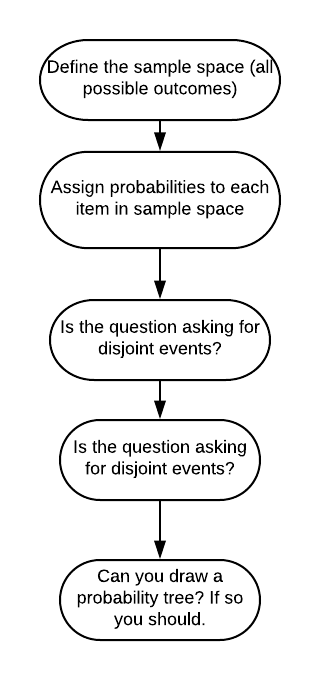Many high-achieving students tell me that they try to visualise what they are dealing with as much as possible.

### Example#

Let’s suppose we roll a 6-sided dice and we want to work out the probability we get a 4.

1. Count the number of possible events. There are 6 sides to the dice. So there are 6 possible events
2. Decide which event you are examining for probability. The problem let’s us know we are trying to roll a four.
3. Count the number of chances that heads can occur out of the possible events. There is only one side of the die that has 4 dots, so there is only 1 chance to roll a four out of 6 total chances.
4. Write the number of chances heads could occur over the number of possible events in a fraction. (1/6)

Although this is a simple problem to solve,it illustrates the important steps to take when solving harder probability problems.

### Events#

Events are often overlooked in probability theory and are not talked about much, so I took it upon myself to expand on what an event is and why they’re important in this section.

An event is a set of outcomes of an experiment in probability. In Bayesian Probability an event is defined as describing the next possible state space using knowledge from the current state.

An event is often denoted with the character ‘e’. Such as the probability being P(e) of an event. Events are a lot more important in probability than most people make them out to be.

An event can be the result of rolling a dice such as a “5” or getting a Tail when flipping a coin.

Events can be:

1. Independent — Each event is not effected by previous or future events.
2. Dependent — An event is affected by other events
3. Mutually Exclusive — Events can’t happen at the same time

### Why are Events Important?#

Well, events allow us to do some pretty amazing things with probability. Take for instance, the Monty Hall Problem. Attempt the question below: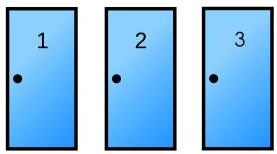One of the doors above contains a fancy sports car, the other 2 doors contain goats. Pick any door you like, go on!

Okay, let’s say you picked #1, the game show host will open a door that contains a goat, so let’s say we open door number 3 and it contains a goat. So you know that door 1 is your pick, door 3 is a goat and door 2 is untouched. Note: It doesn’t matter what door you originally picked, what matters is that you pick a door and the gameshow host opens a door with a goat in it.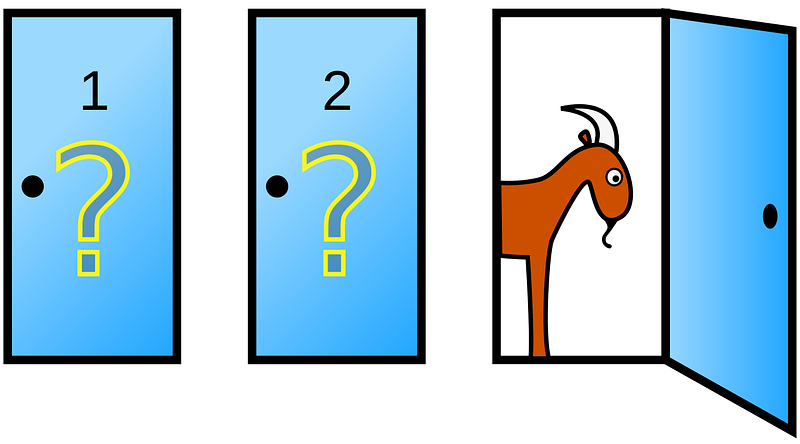The game show then asks: “Are you sure door number 1 is right? Do you want to switch?”

What do you do?

Well, probability states that we should pick door number 2, as in you would switch. Why? well, door number 2 has a 2/3 chance or 77% chance of containing the car and door number 1 (your original pick) has a 33% chance of containing a car.

Whaaaaattt??

This is a famous probability problem called the Monty Hall Problem and it displays how events can affect probabilities. For an explanation of this, watch this Numberphile video below:

### The Probability of the Complement of an Event#

The complement of an event is all the other outcomes of an event.

For example, if the event is Tails, the complement is Heads. If the event is {Monday, Tuesday}, the complement is {Wednesday, Thursday, Friday, Saturday, Sunday}.

If you know the probability of p(x) you can find the compliment by doing 1 — P(x). Since all probabillities equal 100%, we can express this as 1.

### Why is the Complement Useful?#

Sometimes it is easier to work out the complement first before the actual probability. For example:

``````Work out the probability that when 2 die are thrown that the two scores are different
``````

A different score is like getting a 2 and 3, or 1 and 6. The set of all possible different scores is quite large, but the complement of all possible different scores (scores are the same) is quite low. In fact, it is:

{ (1, 1), (2, 2), (3,3), (4,4), (5,5), (6,6) }

The total number of different combinations is 6*6 which is 36, so the probability of getting a score that is the same is 6/36 or 1/6. Now we can take 1/6 away from 1 (think of 1 as the universal set) which equates to 5/6.

### The Union of Two Events (Inlcusion-Exclusion Principle)#

If two events are mutually exlusive (they cannot occur at the same time) then the probability of them happening at the same time is 0.

If two events are not mutually exclusive, then the probability of the union of the two events is the probability of both events added together.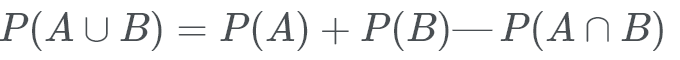The reason we take away the intersection of A and B is because P(A) + P(B) contains all that it is in A or B but because of the way union works, there will be an intersection which will make 2 A’s and 2 B’s so we need to take away the intersection to get the probability of each events.

In other words, A contains elements that are in B and B contains elements that are in A. By adding: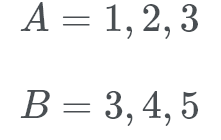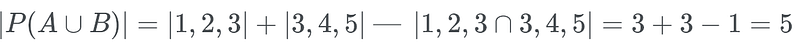### Union of Three Disjoint Events#

Suppose that I were to roll a fair dice 3 times.S is the set of sequences of events over length three such that { 1..6)³}P(x) = 1/666 = 1/216 for all x ∈ SWhat is the probability that we roll at least one 6?So because we throw the dice 3 times, let E1 be the probability that the dice roll is a 6, E2 = P(6), E3 = P(6)We would like to work outP(E1∪E2∪E3)

Remember, the union of probabilities is P(A) + P(B) — Intersection of A and B. We want the union of A, b and C which also includes the intersection in the middle. We take away the intersections of A B, A C, B C and add the intersection of all 3 to get the middle part.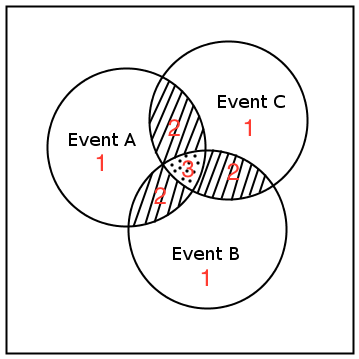So this is just: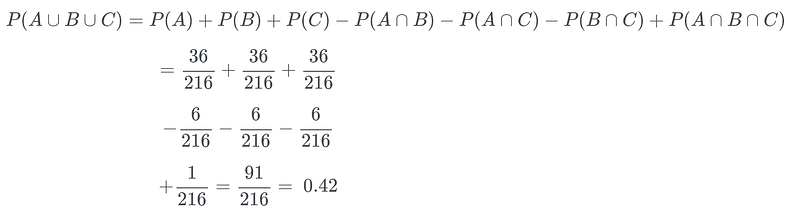You might have noticed that the intersection is 6/216. This may seem confusing because we didn’t hand-define a set for this. Worry not: The formula for intersection is: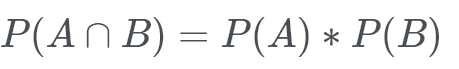### Example Question#

``````Given 4 coins, what is the probability that at least 3 of them come up tails?
``````

The event that at least 3 coins come up tails is the union of five disjoint events, that all coins come up tails (1 disjoint event) and that 4 specified coins (4 disjoint events) come up heads. This may sound confusing, so I’ll explain it visually. Feel free to skip the next paragraph if you’re not confused.

A disjoint event means that events cannot happen at the same time. The first disjoint event is “what if all coins come up tails?” That is that the 5 coins {T, T, T, T, T}. The other 4 events are what if one specified coin comes up heads? So the first disjoint event is {H, T, T, T}, the second is {T, H, T, T} etc. Since we need at least 3 coins to be tails, {H, H, T, T} isn’t valid.

The union of 5 disjoint events is the probability of each event happening added together.

First, lets find out the probability that any probability within this space is possible. The problem space is {H, T} over 4 different coins. Each coin has a 1/2 chance of being heads or tails and there are 4 coins so 1/2 * 1/2 * 1/2 * 1/2 is 1/16 chance of any possible outcome in the state space.

Therefore the probability of an event is P(1/16)

Know that we know how likely it is to get any combination of {H, T} over the 4 coins we can use this to work out how likely it is to get the 5 disjoint events. Since each event is disjoint, one event does not effect another so it’s just a case of 1/16 * 5 (for the 5 disjoint events) which results in 5/16.

Thus the probability of at least 3 coins coming up tails is 5/16.

### Conditional Probability#

Conditional probability is where an event can only happen if another event has happened. Let’s start with an easy problem:

John’s favourite programming languages are Haskell and x86 Assembley. Let A represent the event that he forces a class to learn Haskell and B represent the event that he forces a class to learn x86 Assembley.On a randomly selected day, John is taken over by Satan himself, so the probability of P(A) is 0.6 and the probability of P(B) is 0.4 and the conditional probability that he teaches Haskell, given that he has taught x86 Assembley that day is P(A|B) = 0.7.Based on the information, what is P(B|A), the conditional that John teaches x86 Assembley given that he taught Haskell, rounded to the nearest hundredth?

The probability of P(A and B) = P(A|B) * P(B) read “|” as given, as in, “A|B” is read as “A given B”. It can also be written as P(B|A) * P(A).

The reason it is P(A|B) * P(B) is because given the probability of “Given the probability that B happens, A happens” and the probability of B is P(B). (A|B) is a different probability to P(B) and P(A and B) can only happen if P(B) happens which then allows P(B|A) to happen.

So we can transform this into a mathematical formula:

P(A and B) = P(A|B) * P(B) = 0.7 * 0.5 = 0.35Solving itP(B|A) * P(A)P(A) = 0.5So0.6 * P(B|A)Now we don’t know what P(B|A) is, but we want to find out. We know that P(B|A) must be a part of P(A and B) because P(A and B) is the probability that both of these events happen so…P(A and B) = 0.350.35 = P(B|A) * 0.5With simple algebraic manipulation0.35/0.5 = P(B|A)P(B|A) = 0.7

For a visual explanation of conditional probability, watch this video by Khan Academy

### Bayes Therom#

Bayes Therom allows us to work out the probability of events given prior knowledge about the events. It is more of an observation than a therom, as it correctly works all the time. Bayes therom is created by Thomas Bayes, who noted this observation in a notebook. He never published it, so he wasn’t recgonised for his famous therom during his life time.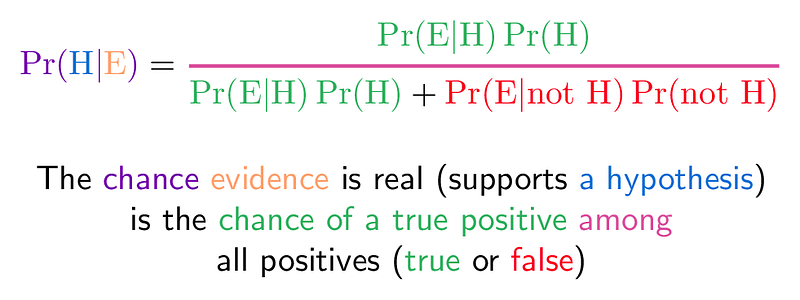Bayes Therom from https://betterexplained.com/articles/colorized-math-equations/ The probability of A given B is the probability of B given A (note: it’s reversed here) times by the probability of A divided by the probability of B.

Of course this sounds confusing, so it may help to see an example.

Suppose a new strand of mexican black tar heroin is found on the streets and the police want to identify whether someone is a user or not.The drug is 99% sensitive, that is that the proportion of people who are correctly identified as taking the drug.The drug is 99% specific, that is that the proportion of people who are correctly identified as not taking the drug.Note: there is a 1% false positive rate for both users and non users.Suppose that 0.5% of people at John Moores takes the drug. What is the probability that a randomly selected John Moores student with a positive test is a user?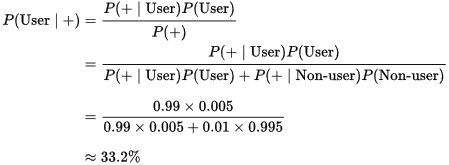Once you have all the information, it’s simply a case of substituting in the values and working it out.

Below is a video explaining Bayes Therom intuitively with real-world examples along with the history behind it as well as the philosophy of Bayes Therom:

If you want to see how Bayes Therom is used in Machine Learning — check this out!

### Random Variables#

A random variable is a function, it is not random or a variable.

A random variable does not need to specify the sample space S directly but assign a probability that a variable (X) takes a certain value. Unlike previous probability where we needed to define a sample space, we only care about the probability itself.

Random variables are often written as P(f=r) where f is the event name and r is the probability.

The probably has to be between 0 and 1, like all probability values.

We write NOT (using any notation you may wish) (F=r) for the event that F is every variable apart from R.

An example of this

``````P(Die=1) = 1/6The probability that this die takes the value 1 is 1/6NOT P(Die=1) is the event that the die is(Die=2) OR (Die=3) OR (Die=4) OR (Die=5) Or (Die=6)
``````

The complement of P(f=r) ; the notation used to represent random variables, is 1 — P(f=r), where 1 is 100% or just 1.

We sometimes use symbols (words) instead of numbers to represent random variables. This is really useful. Let’s say the weather can be 1 of 4 states, sunny, rain, cloudy, snow. Thus, instead of assigning Weather = 1 we could write Weather = sunny.

Sometimes it lengthy to write up all the probabilities such as P(Weather = sunny) = 0.7 or P(Weather = rain) = 0.3. If the values are fixed in order then we could write P(Weather) = (0.7, 0.3)

We use bold-face P to indicate that the result is a vector of numbers representing the individual values of Weather. An example of this is: P(Weather) = (0.7, 0.3).

### Joint Probability Distributions#

A joint probability distribution allows you to have multiple random variables, typically 50 or 100 but our examples will include fewer.

A possible joint probability distribution P(Weather, Cavity) for the random variables Weather and Cavity is given by the following table: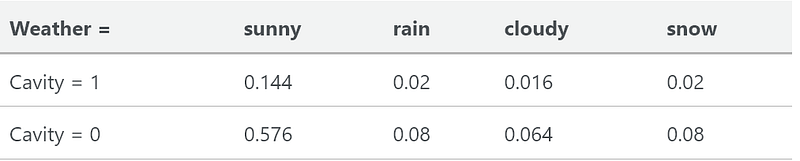This is a joint probability distribution for tooth cavities and the weather. Cavity is a boolean value, it is either 0 or 1 and there are 4 options for the weather. If we want to create a joint probability distribution of P(Weather, Cavity) we would make the table above.

The probability for weather = sunny, and cavity = 1 is 0.144. The probability of the joint distribution sums to 1.

### Full Joint Probability Distribution#

We call it a full joint probability distribution if everything that is relevant in the domain is included. Unlike the above example, cavities and weather aren’t in the same domain.

Assume the random variables Toothache, Cavity, Catch fully describe a visit to a dentist

Then a full joint probability distribution is given by the following table: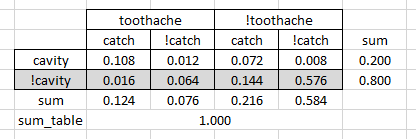From here

### Marginalisation#

One can computer the marginal probabilities of random variables by summing the variables. For example in the above example, if one wanted to sum the probability of P(Cavity=1) then you will sum all the probabilities where the cavity is equal to 1.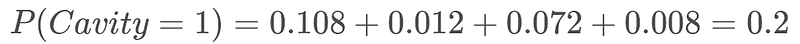### Conditonal / Posterior Probability#

We can calculate the conditional / posteior probability of a full joint distribution the same way we would do it normally.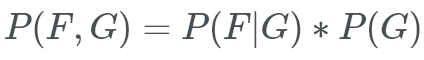Note that (F, G) signifies F (and, intersection) G.

### Expected Value#

The expected value is exactly what it sounds like, what do you expect the value to be? You can use this to work out the average score of a dice roll over 6 rolls, or anything really relating to probability where it has a value property.

Given the outcomes=(1, 2) and the probabilities=(1/8, 1/4) the expected value, E[x] is E[x] = 1(1/8) + 2(1/4) = 0.625.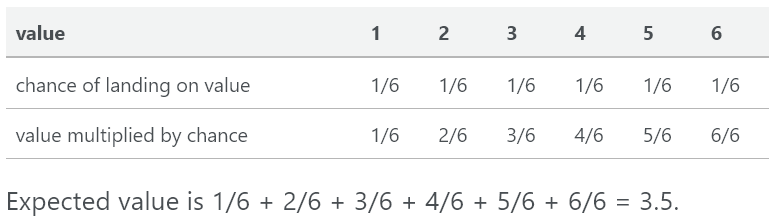Suppose we’re counting types of bikes, and we have 4 bikes. We assign a code to each bike like so: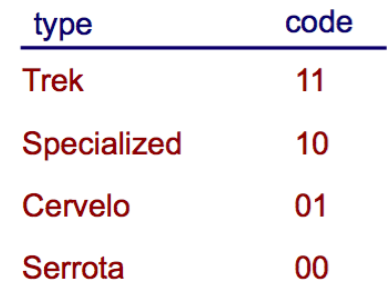For every bike, we give it a number. For every coding, we can see we use 2 bits. Either 0 or 1. For the expected value, not only do we need the value for the variable but the probability. Each bike has equal probability. So each bike has a 25% chance of appearing.

Calculating the expected value we multiply the probability by 2 bits, which gets us: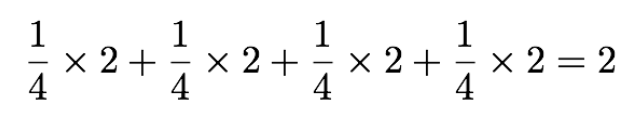What if the probability wasn’t equal?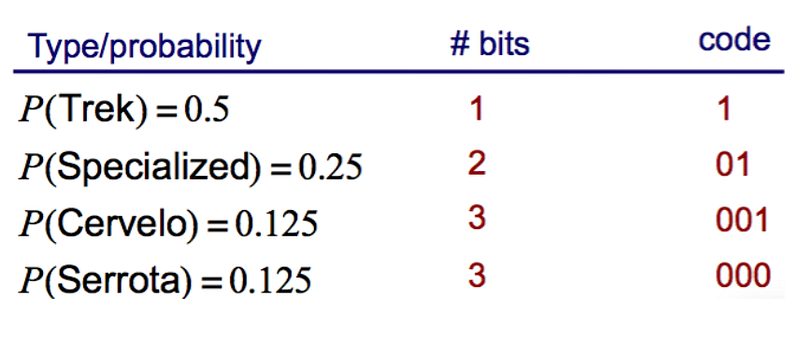What we need to do is to multiply the number of bits by the probability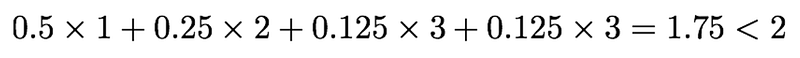### Entropy#

Entropy is a measure of uncertainty assiocated with a random variable. It’s defined as the expected number of bits required to communicate the value of the variable.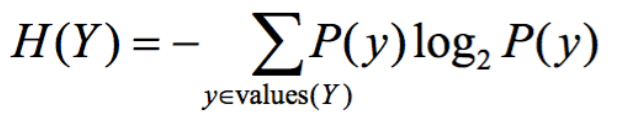Entropy is trying to give a number to how uncertain something is.

### Statistics#

Statistics is not probability theory. Statistics is the real world application of ideas which come from probabiltiy theory. These can invole:

1. Psepholohy — Analysing voting patterns
2. Data analysis — Data science
3. Quality control

### Sample Space#

A sample space is a collection of data as a single finite set that looks something like: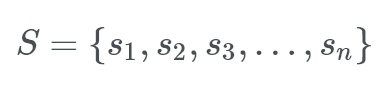Where S is the sample space.

### Probability Distribution#

Let’s say we want to pick a random person from a set of all people who read the Sun newspaper. The probability of a single person being chosen is: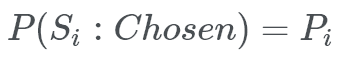A probability distribution is a sample space where every item has a probability value between 0 and 1 assigned to them that represents how likely they are to be picked.

In total, if s is an element of S, that is, if an element s is a part of the set (group) of sample space, S, then: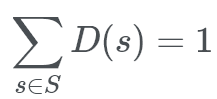If you add the probability of every element in the sample space, it has to sum to 1.

When we want to sample this data set, we could just go through every single person in the dataset to get a good feel of the generalness of this sample. However, if there were 7 billion people in this dataset that may take a very very long time.

There are 2 ways we can now sample the data.

We can either randomly choose people from the dataset and use that as our sample or we can hand-pick a specific subset of the data to use.

A uniform dataset is one where everyone is equally likely to be picked. A biased sample is not uniform, the people were hand picked.

Unbiased data sets seem “fair” whereas unbiased seem “unfair”. With an unbiased sample we cannot fix the outcome. We cannot change the data to our favor.

Sometimes we don’t care about “fairness” and sometimes unbiased samples can lead to unexpected results.

### Random Variables#

Remember earlier when we said that random variables are functions? Well, if you apply a random variable to a sample space, a population like so: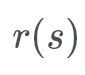You get a biased data set from that sample space. It’s biased because we’re not randomly picking people in the set; we are applying a filter — a rule to the set to get a subset of the population.

The notion of a probability distribution. This is the description of the probability that a member of a population (ie set) is selected. For example if we consider a single die the population has 6 members: {1,2,3,4,5,6} We might have a probability distribution corresponding to a fair die so that each has a probability of 1/6 of being chosen. If it’s a biased die then, for example, the probability distribution could be P=5/6 P=0 and P=P=P=P=1/24 With this the sum of individual outcomes is 1.

A random variable is best thought of firstly by forgetting about probabilities and thinking of an arbitrary function from the population to for instance the real numbers. In the die example we could choose f(x)=x² now unlike the probability distribution function the function chosen has no constraints: members of the population do not have to have values between 0 and 1, the sum of the function values doesn’t have to add up to 1. Where the idea of “random variable” enters is when a function is combined with a probability distribution. Now the distribution is treated not as simply choosing a MEMBER of the population but as choosing the VALUE of the function in a random style, that is instead of returning the selected member (eg result of throwing a die) the function value for that member is reported (eg the square of the number thrown).

### Mean Average Value with Random Variables#

Given a population, S, whose members are sampled according to a distribution, D. The mean (expected) value of the random variable r(s) under D is denoted as

This is simply states that the expected value is a “weighted” sum (taken over all the members, s, of the total population, S) of: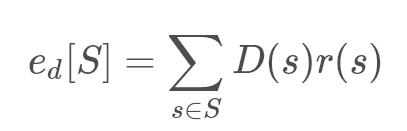*the chance that D selects s multipled by the value of the function returned by r for s, ie r(s).*In Unbiased Distributions

#### Unbiased Distributions#

In unbiased distributions the expected value is just the total sum of all the random variables divided by the population size: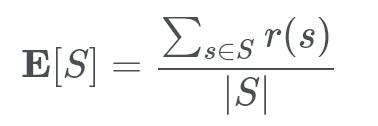This is just your typical mean value, the one you learn in school. My teacher taught me a cool song to remember the differences between mean, range, median etc.

Hey diddle diddle the median is the middle we add and divide for the mean. The mode is the one you see the most, and the range is the difference in between!

Suppose S is a collection of outcomes that may appear by rolling a die 6,000 times.

Then for a “fair” die you would expect to see each outcome 1,000 times.

Suppose we have a game where players stake £1 and if the die lands on one of {1, 2, 3} the player gets £2 afterwards otherwise they lose their stake. In a fair game the player can expect to win 3/6 = 1/2 = half of the time.

### Confidence Testing#

Let’s say the hypothesis of an experiment’s outcome is X, and the actual outcome is Y.

The outcome Y is so far away from the prediction that the hypothesis is false. This is called significance.

A null hypothesis states that the outcome will be X.

Significance represents that the likelihood of the observed outcome being “consistent” with the predicted outcome.

An hypothesis can be “rejected” with observed outcomes with three increasing levels of confience:

1. The probability that X holds given Y is at most 0.05 (significant)
2. The probability that X holds given that Y has resulted is at most 0.01 (highly significant)
3. The probability that X holds given that Y has resulted is 0.001 (very highly siginificant)

There are two types of errors that can occur here:

Type 1 error — A true hypothesis is rejected Type 2 error — A false hypothesis is accecpted

### Measuring Signifcance#

The outcome of the event will get “closer and closer” towards the expected value can be expressed as a formula called the deviance. Recall that the event of a random variable in a sample space is: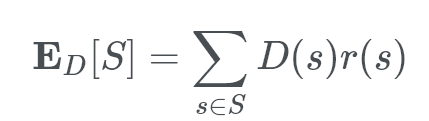Variance is just:

“How far away a chosen member is from the expected variable”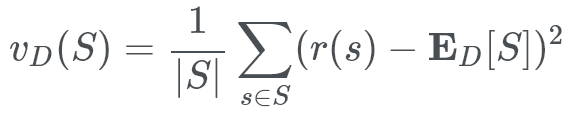Doesn’t that look horrible? Well, if we were to put the first formula in it would look like: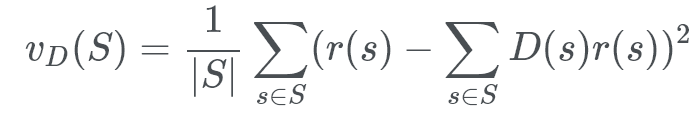Doesn’t that look like the most horrible formula ever?

the r(s) part is the random variable, the subset of the population. The part is the expected value of a random member.

Variance always produces a non negative value.

The standard deviation is just this formula, square rooted.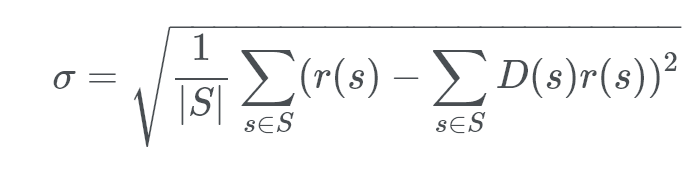It’s actually more commonly written as:

I just wanted to see how convuluted the formula could become.

The standard deviation is just:

“How far away the largest (or smallest) data point is from the mean average”.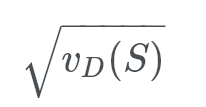### Q-test#

Given a predicted outcome, X, of an experiment and the actual outcome, Y. If we know the standard deviation for the environment in which the experiment is set, then we can compute the value: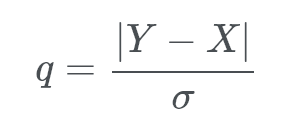If q > 0.01 then X holds with probability at best 0.05 If q > 2.33 then X holds with probability at best 0.01 If q > 3.09 then X holds with probability at best 0.001